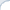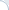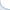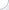Preamble: Hongya Wang and colleagues have written a thought-provoking paper where they argue that performance analysis of a popular method: a Locality Sensitive Hashing (LSH) has a fatal flaw . Here is my take on this: even though their logic seems to be correct, I do not fully agree with their conclusions. In what follows, I consider this issue in more detail. See also my note here and a discussion in the blog of Daniel Lemire.

A locality-sensitive hashing, commonly known as LSH, is a popular method to compute locality-sensitive, distance-aware, hash values. More specifically, given two objects $x$ and $y$, the probability that their locality-sensitive hash values $h(x)$ and $h(y)$ are the same, depends on the distance between $x$ and $y$. The smaller is the distance, the more likely $x$ and $y$ collide with respect to the hash function $h()$. An event of getting $x$ and $y$ such that $h(x)=h(y)$ is called a collision.

This kind of distance sensitivity is a super-useful property, which fuels many probabilistic algorithms including approximate counting, clustering, and searching. In particular, with a good choice of a locality-sensitive function, we can build a hash table where close objects tend to be in the same bucket (with a sufficiently high probability). Thus, elements stored in the same bucket are good candidates for placing in the same cluster. In addition, given a query object $q$, potential nearest neighbors (again with some probability) can be found in the bucket corresponding to the hash value $h(q)$. If a collision probability for a single hash function is low, we need to build several hash tables over the same set. If we miss the nearest neighbor in one hash table, we still have a chance to find it another one. The more hash tables we build, the higher is a probability of success.2 Clearly, we need a family of locality-sensitive hash functions, but how do we get one?

In one specific, but important, case, we deal with bit (i.e., binary) vectors where dissimilarity is computed using the Hamming distance (the distance between bit vectors $x$ and $y$ is equal to the number of non-matching bits). In this setup, we can "create" binary hash functions by simply taking the value of an i-th bit. One can see that this is a projection to the 1-dimensional sub-space. The number of such functions $h^i()$ is equal to the length of a bit vector. Formally, the hash function number $i$ is defined as: $h^i(x) = x_i$.

This function is locality sensitive: The larger is the number of matching bits between vectors, the higher is the probability that they have equal i-th bits. What is the exact probability of a collision in this case? Typically, it is claimed to be $\frac{n - d}{n}$, where $n$ is the length of vectors and $d$ is the Hamming distance. For example, if vectors differ only in one bit, there is apparently $n$ ways to randomly select a projection dimension and, consequently, $n$ ways to select a hash function. Because, vectors differ only in a single bit number, only one choice of the hash function produces non-matching values. With respect to the remaining hash function, both vectors will collide. Thus, the collision probability is $\frac{n-1}{n}$.

Here is a subtle issue. As noted by Hongya Wang et al. , we do not randomly select a hash function for a specific pair of objects. Rather, we select hash functions randomly in advance and only afterwards we randomly select objects. Thus, we have a slightly different selection model than the one used in the previous paragraph! Using simple math, one can verify that if the set of objects comprises all possible bit vectors (and these bit vectors are selected randomly and uniformly), the two selection models are equivalent.

However, in general, these selection models are not equivalent. Consider a data set of bit vectors, such that their n-th bit is always set to one. For the first $n-1$ bits, all combinations are equally likely. Note that we essentially consider an (n-1)-dimensional sub-space with equi-probable elements. In this sub-space, two selection models are equivalent. Thus, a probability of a collision is $\frac{n - 1 - d}{n-1}$.

Note that this is true only for the first $n-1$ hash functions. Yet, for the function $h^n()$ the probability of a collision is different, namely it is one! Why? Because, we have a biased data set, where the value of the last (n-th) bit in each vector is always equal to one. Hence, a projection to the n-th dimension always results in a collision (for any pair of objects).

Hongya Wang et al.  go even further and carry out simulations. These simulations support their theoretical observations: The probabilities obtained via two different selection models are not always the same. So, it looks like the analysis of the LSH methods does rely on the simplifying assumption: The probability of a collision can be computed assuming that a hash function is randomly selected while the objects are fixed. Yet, I think that this simplification does not make a big difference, and it is the paper by Hongya Wang and colleagues that makes me think so.

First, even though collision ratios obtained via simulations (see Table 1 and Table 2 in the paper ) are sometimes quite different from predicted theoretical values, most of the time they are close to the theoretical predictions (again, based on the assumption that a hash function is selected randomly while objects are fixed). Second, as shown in Theorem 1, the average collision ratio rarely diverges from the theoretical value, if the number of objects and hash functions is large (which is mostly true in practice). Finally, I do not think that data sets where a significant fraction of the objects collide with respect to a given locality-sensitive hash function are likely. Such "mass" collisions might be a concern in some adversarial scenario (e.g., in the case of a DOS attack), but I would not expect this to happen under normal circumstances.

One nice property of the LSH is that we can tune parameters of the method to achieve a certain level of recall. Furthermore, these parameters can be selected based on the distribution of data . Thus, it would be possible to retrieve a true nearest neighbor in, e.g., about 50%, of all searches (in the shortest possible time). What happens if we miss the true answer? It is desirable that we still get something close. Yet, it turns out, that we often get results that are far from the nearest neighbor. This observation stems primarily from my own experiments , but there other papers where authors came to similar conclusions .

Thus, it may not be always possible to rely solely on the LSH in the nearest neighbor search. Streaming first story detection is one example, where authors use a hybrid method, in which an LSH-based search is a first step . If the LSH can find a tweet that is reasonably close to the query, the tweet topic is considered to be repetitive (i.e., it is not new). However, if we fail to find a previously created close-topic tweet using the LSH, we cannot be sure that the close-topic tweet does not exist. Why? The authors surmise that this may happen when the answer is not very close to the query. However, it might also be because the LSH tend to return a distant tweet even if a close-topic tweet exists in the index. To eliminate such potential false negatives, the authors use a small inverted file, which is built over a set of recent tweets.

To summarize, LSH is a great practical method based on reasonable theoretical assumptions, whose performance was verified empirically. It is possible to tune the parameters so that a certain recall level is achieved. Yet, there is seemingly no control in regard to how far are the objects in a result set from true nearest neighbors, when these true neighbors elude the LSH search algorithm.

1) This is a bit simplified description. In the real LSH, a hash function is often built using several elementary and binary hash functions, each of which is locality sensitive. In the case of real-valued vectors, one common approach to obtain binary functions is through a random projection.